# Gravitation.pptx

1 Jun 2023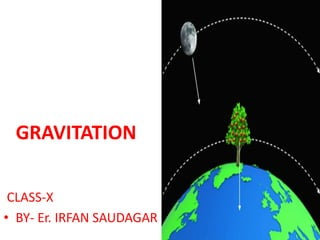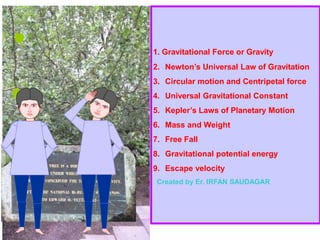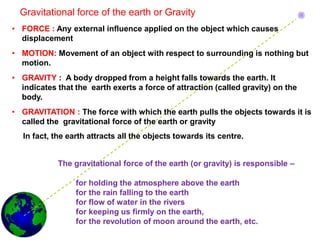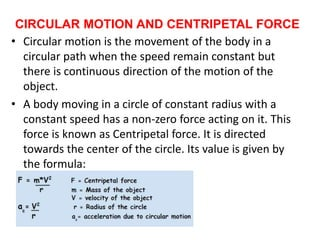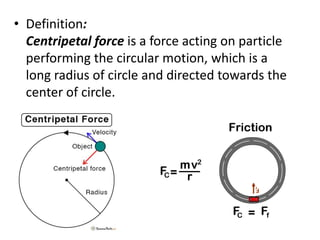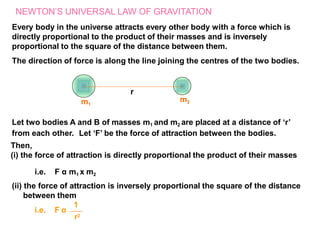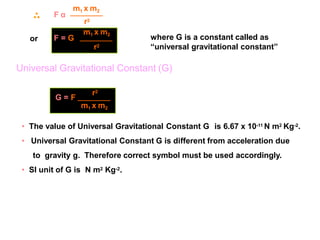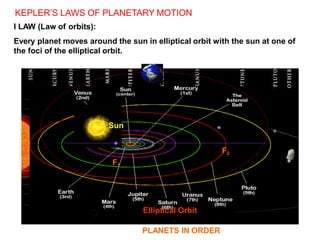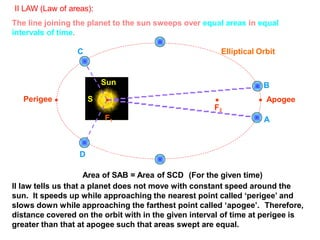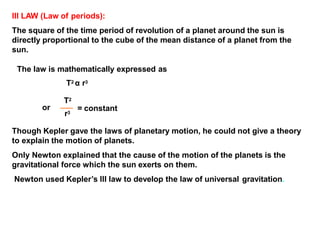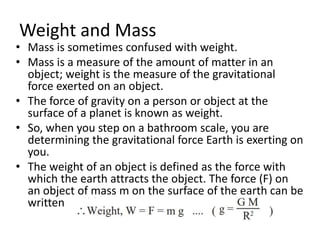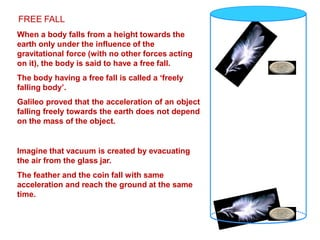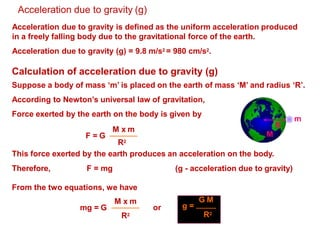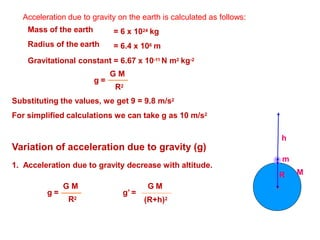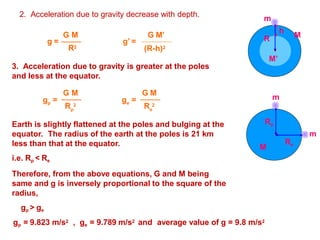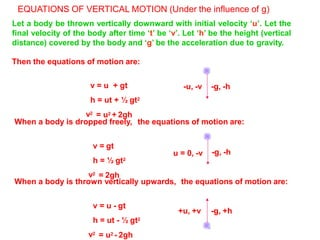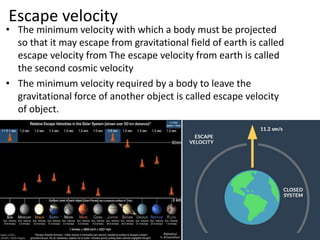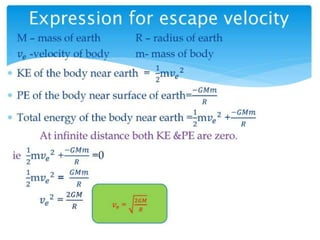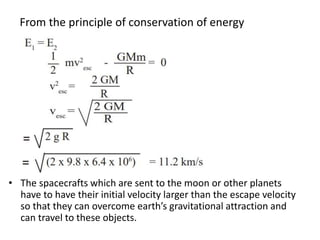1 sur 19

### Gravitation.pptx

• 2. 1. Gravitational Force or Gravity 2. Newton’s Universal Law of Gravitation 3. Circular motion and Centripetal force 4. Universal Gravitational Constant 5. Kepler’s Laws of Planetary Motion 6. Mass and Weight 7. Free Fall 8. Gravitational potential energy 9. Escape velocity Created by Er. IRFAN SAUDAGAR
• 3. Gravitational force of the earth or Gravity • FORCE : Any external influence applied on the object which causes displacement • MOTION: Movement of an object with respect to surrounding is nothing but motion. • GRAVITY : A body dropped from a height falls towards the earth. It indicates that the earth exerts a force of attraction (called gravity) on the body. • GRAVITATION : The force with which the earth pulls the objects towards it is called the gravitational force of the earth or gravity In fact, the earth attracts all the objects towards its centre. The gravitational force of the earth (or gravity) is responsible – for holding the atmosphere above the earth for the rain falling to the earth for flow of water in the rivers for keeping us firmly on the earth, for the revolution of moon around the earth, etc.
• 4. CIRCULAR MOTION AND CENTRIPETAL FORCE • Circular motion is the movement of the body in a circular path when the speed remain constant but there is continuous direction of the motion of the object. • A body moving in a circle of constant radius with a constant speed has a non-zero force acting on it. This force is known as Centripetal force. It is directed towards the center of the circle. Its value is given by the formula:
• 5. • Definition: Centripetal force is a force acting on particle performing the circular motion, which is a long radius of circle and directed towards the center of circle.
• 6. NEWTON’S UNIVERSAL LAW OF GRAVITATION Every body in the universe attracts every other body with a force which is directly proportional to the product of their masses and is inversely proportional to the square of the distance between them. The direction of force is along the line joining the centres of the two bodies. r m1 m2 i.e. F α 1 r2 Let two bodies A and B of masses m1 and m2 are placed at a distance of ‘r’ from each other. Let ‘F’ be the force of attraction between the bodies. Then, (i) the force of attraction is directly proportional the product of their masses i.e. F α m1 x m2 (ii) the force of attraction is inversely proportional the square of the distance between them
• 7. or F α m1 x m2 r2 where G is a constant called as “universal gravitational constant” m1 x m2 F = G r2 G = F r2 m1 x m2 Universal Gravitational Constant (G) • The value of Universal Gravitational Constant G is 6.67 x 10-11 N m2 Kg-2. • Universal Gravitational Constant G is different from acceleration due to gravity g. Therefore correct symbol must be used accordingly. • SI unit of G is N m2 Kg-2.
• 8. KEPLER’S LAWS OF PLANETARY MOTION I LAW (Law of orbits): Every planet moves around the sun in elliptical orbit with the sun at one of the foci of the elliptical orbit. F1 F2 Sun Elliptical Orbit PLANETS IN ORDER
• 9. F1 F2 Sun S II LAW (Law of areas): The line joining the planet to the sun sweeps over equal areas in equal intervals of time. C Elliptical Orbit B Apogee A Perigee D Area of SAB = Area of SCD (For the given time) II law tells us that a planet does not move with constant speed around the sun. It speeds up while approaching the nearest point called ‘perigee’ and slows down while approaching the farthest point called ‘apogee’. Therefore, distance covered on the orbit with in the given interval of time at perigee is greater than that at apogee such that areas swept are equal.
• 10. Though Kepler gave the laws of planetary motion, he could not give a theory to explain the motion of planets. Only Newton explained that the cause of the motion of the planets is the gravitational force which the sun exerts on them. Newton used Kepler’s III law to develop the law of universal gravitation. = constant r3 III LAW (Law of periods): The square of the time period of revolution of a planet around the sun is directly proportional to the cube of the mean distance of a planet from the sun. The law is mathematically expressed as T2 α r3 T2 or
• 11. Weight and Mass • Mass is sometimes confused with weight. • Mass is a measure of the amount of matter in an object; weight is the measure of the gravitational force exerted on an object. • The force of gravity on a person or object at the surface of a planet is known as weight. • So, when you step on a bathroom scale, you are determining the gravitational force Earth is exerting on you. • The weight of an object is defined as the force with which the earth attracts the object. The force (F) on an object of mass m on the surface of the earth can be written
• 12. FREE FALL When a body falls from a height towards the earth only under the influence of the gravitational force (with no other forces acting on it), the body is said to have a free fall. The body having a free fall is called a ‘freely falling body’. Galileo proved that the acceleration of an object falling freely towards the earth does not depend on the mass of the object. Imagine that vacuum is created by evacuating the air from the glass jar. The feather and the coin fall with same acceleration and reach the ground at the same time.
• 13. Acceleration due to gravity (g) Acceleration due to gravity is defined as the uniform acceleration produced in a freely falling body due to the gravitational force of the earth. Acceleration due to gravity (g) = 9.8 m/s2 = 980 cm/s2. Calculation of acceleration due to gravity (g) Suppose a body of mass ‘m’ is placed on the earth of mass ‘M’ and radius ‘R’. According to Newton’s universal law of gravitation, Force exerted by the earth on the body is given by R m M F = G M x m R2 This force exerted by the earth produces an acceleration on the body. Therefore, F = mg (g - acceleration due to gravity) From the two equations, we have mg = G M x m R2 or G M g = R2
• 14. Acceleration due to gravity on the earth is calculated as follows: Mass of the earth Radius of the earth = 6 x 1024 kg = 6.4 x 106 m g = Gravitational constant = 6.67 x 10-11 N m2 kg-2 G M R2 Substituting the values, we get 9 = 9.8 m/s2 For simplified calculations we can take g as 10 m/s2 Variation of acceleration due to gravity (g) 1. Acceleration due to gravity decrease with altitude. h M m R g = R2 g’ = G M G M (R+h)2
• 15. 2. Acceleration due to gravity decrease with depth. g = R2 g’ = G M G M’ (R-h)2 h m 3. Acceleration due to gravity is greater at the poles and less at the equator. p e g = g = G M G M p e R 2 R 2 m M Rp m Re Earth is slightly flattened at the poles and bulging at the equator. The radius of the earth at the poles is 21 km less than that at the equator. i.e. Rp < Re Therefore, from the above equations, G and M being same and g is inversely proportional to the square of the radius, gp > ge gp = 9.823 m/s2 , ge = 9.789 m/s2 and average value of g = 9.8 m/s2 M M’ R
• 16. EQUATIONS OF VERTICAL MOTION (Under the influence of g) Let a body be thrown vertically downward with initial velocity ‘u’. Let the final velocity of the body after time ‘t’ be ‘v’. Let ‘h’ be the height (vertical distance) covered by the body and ‘g’ be the acceleration due to gravity. Then the equations of motion are: v = u + gt h = ut + ½ gt2 v2 = u2 + 2gh When a body is dropped freely, the equations of motion are: v = gt h = ½ gt2 v2 = 2gh When a body is thrown vertically upwards, the equations of motion are: v = u - gt h = ut - ½ gt2 v2 = u2 - 2gh -u, -v -g, -h u = 0, -v -g, -h +u, +v -g, +h
• 17. Escape velocity • The minimum velocity with which a body must be projected so that it may escape from gravitational field of earth is called escape velocity from The escape velocity from earth is called the second cosmic velocity • The minimum velocity required by a body to leave the gravitational force of another object is called escape velocity of object.
• 19. From the principle of conservation of energy • The spacecrafts which are sent to the moon or other planets have to have their initial velocity larger than the escape velocity so that they can overcome earth’s gravitational attraction and can travel to these objects.